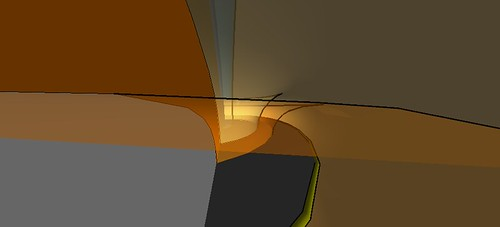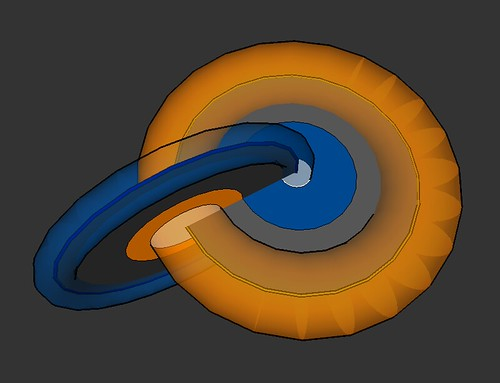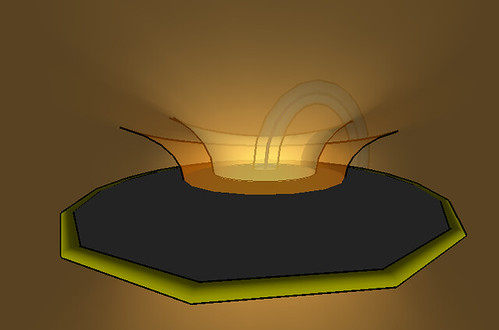## Toroidal Coordinates on S^3We’re looking at what amounts to being the genus 1 Heegaard sweep-out of S^3. There are a few concentric tori, two core curves, and two intersecting meridional disks. Of course above we’re looking at an odd perspective from the level of an orangish torus.

Pulling back, just looking at the two core curves and a couple of disks they bound, we see the following.Since$\mathbb{S}^3$ is the unit sphere in$\mathbb{C}^2$, we can think of these two disks as the unit$z$–disk and the unit$w$–disk. You might think of each of these in terms of polar coordinates:$r e^{i \theta}$. Then the angles$\theta_z$ and$\theta_w$ are independent while the two radii are bound by$r_z^2 + r_w^2 =1$. In some sense this gives what one might call toroidal coordinates on$\mathbb{S}^3$.This is one framework for realizing the knots and curves that come from links of singularities (such as the trefoil coming from$z^2 w^3 = 1$) and visualizing knots from grid diagrams among others.

Just because I could, I made a quick fly through. (You might have to run it a second time if it races past you. Still trying to figure out how to loop.)

And here are some more stills. Yet more are on my Flickr.~ by Ken Baker on August 29, 2008.

### 4 Responses to “Toroidal Coordinates on S^3”

1. Really nice, I like the appearance of the generic cusps in the center picture above. In a knotted surface type diagram the two coordinate disks have a unique double point in 4-space: C x C. This seems to occur at the intersection point of the orange and blue annuli in your second diagram.

Are you drawing this, or taking a parameterization and then projecting?

2. You didn’t (less than a href equal quote URL close quote greater than flicker account less than forward slash a greater than your flickr account.

3. Thanks Scott. Yeah I’m just drawing this in SketchUp. Everything here is actually in$R^3$ since that’s the geometry SketchUp uses. So all the tori are circles swept around circles, the disks are round disks.

What would be really neat is if some one could write, say, a ruby script for SketchuUp that facilitated drawing in spherical or hyperbolic space.

I get the distorted views by changing the field of view to around 120 degrees rather than the more standard 35ish degrees.

The model is available here.

Let me fill in some details for others:
That intersection point between the orange and blue annuli occurs at the radius$1/\sqrt{2}$ on each of the disks. The parametrization of the radial coordinates is not standard since we’re requiring$r_z^2+r_w^2 =1$. The only true intersection of the unit disks as they sit in$\mathbb{C} \times \mathbb{C}$ does not appear here since there$r_z^2 + r_w^2 = 0$.

(BTW, clicking on any of these pictures will take you to my flickr acct.)

4. […] circle about the origin of radius for you get a torus separating into two solid tori as in an earlier post. At each and you get just a […]